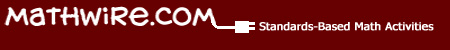More Place Value Activities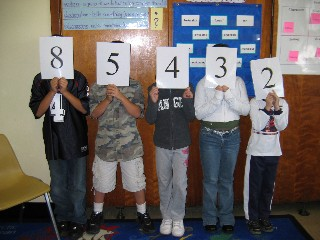## Number Line-Up

This activity is designed to actively involve students in using place value concepts to build numbers.

Objective:   LWDAT use place value to form 3-6 digit numbers to satisfy different conditions.

Materials:

•Demo digit cards, one set for class use
•Small digit cards, one set for each student

Directions:

• Select 3-6 students and give each student one of the demo digit cards.
• Each student selected moves to the front of the classroom and holds the demo digit card in front of them so that classmates can easily see the numeral.
• Students at their desks should select the same digit cards from their decks and put the other cards aside.
• Say a 3-6 digit number aloud, depending on the level of your students.
• Students with the demo digit cards must arrange themselves to create that number.   Students at desks must use their small digit cards to create the dictated number.
• Ask students to say the number in words.
• Ask students to point to the digit in the hundreds place, the tens place, etc.   Students holding cards in the front of the classroom should raise the correct card.
• Repeat this activity a few times to assess student proficiency with basic place value concepts, changing the digits and selecting different students to hold cards at the front of the classroom.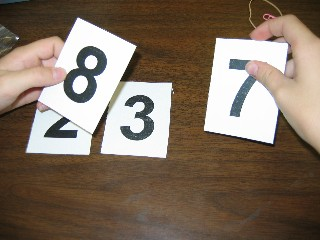## Build a Number

Materials:

•Demo digit cards, one set for class use
•Small digit cards, one set for each student

Directions:

• Identify the digits students will use by handing demo digit cards to selected students (e.g. 3, 5, 8).   Students at desks should take these same digits from their card decks.
• Give directions for students to form a number to meet specific criteria. Students in front rearrange themselves to build a number.   Students at desks arrange digit cards to build a number to satisfy the conditions.
• Build the largest number you can.
• Build the smallest number you can.
• Build a number less than 800.
• Build a number greater than 800.
• Build a number that is between 300 and 500.
• Build a different number that is between 300 and 500.
• Repeat with different digits and different directions.
• Enrichment:   As students develop proficiency with this activity, select a student to give directions for building each number to encourage the use of higher-order thinking skills.
• Center Activities:
• Ask students to play as partners with one partner dictating the number and the other partner arranging the digit cards.   Provide sample number cards, if needed, to prompt the dictation.
• Provide copies ofBuild a Number Worksheet for students to use to create their own versions of this activity.   Students should select the digits to be used and then write 5-10 Build a number conditions.   Students should create answer keys on the back of their papers.   Use these student-generated activities for future place-value practice.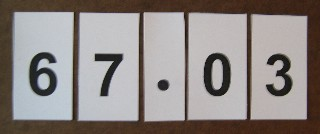## Decimal Build a Number:

Materials:

•Demo digit cards, one set for class use
•Small digit cards, one set for each student

Directions:

• Hand out the decimal point demo card as well as several digit cards to students. (e.g. 7, 2, 0, 4, and decimal point.)
• Students at desks also need a decimal card in addition to these same digit cards.
• Give directions for students to build a number to meet specific criteria. Students in front rearrange themselves to build a number.   Students at desks arrange digit cards to build a number to satisfy the conditions.
• Build the largest number you can.
• Build the smallest number you can.
• Build a number less than 70.
• Build a number greater than 400.
• Build a number that is less than 400.
• Build a number less than 4.
• Build a number that is between 40 and 70.
• Build a different number that is between 40 and 70.
• Repeat with different digits and different directions.
• Enrichment:   As students develop proficiency with this activity, select a student to give directions for building each number to encourage the use of higher-order thinking skills.
• Center Activities:
• Ask students to play as partners with one partner dictating the number and the other partner arranging the digit cards.   Provide sample number cards, if needed, to prompt the dictation.
• Provide copies ofBuild a Number Worksheet for students to use to create their own versions of this activity.   Students should select the digits to be used and then write 5-10 Build a Number conditions.   Students should create answer keys on the back of their papers.   Use these student-generated activities for future place-value practice.## Place Value Games

Games provided extended place value practice for students and allow them to use their conceptual understanding to develop appropriate strategies to win the game.   The best games encourage students to try out many options in search of the best solution.   This search for the best solution prompts additional practice in a highly-motivational setting.

Online directions forHigh-Number Toss Game

Online directions forHigh-Number Toss Game:   Decimal Version

DownloadHigh-Number Toss Recording Sheet for student use in sheet protector with dry erase markers: single round version

DownloadHigh-Number Toss Recording Sheet: 4-round version

PlayPlace Value Puzzler Game online.

PlayPlace Value Game online.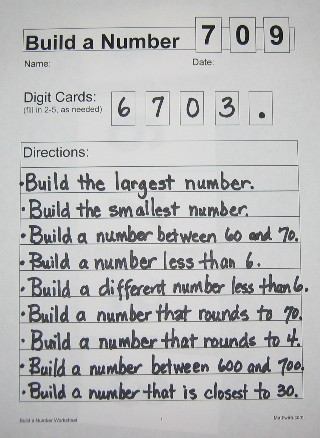• DownloadLargest Sum
• DownloadSmallest Sum
• DownloadLargest Difference
• DownloadSmallest Difference